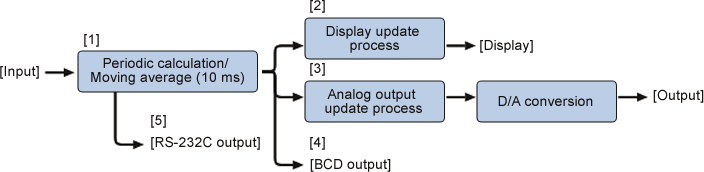Tachometer FAQThe relation of the TM-3100 display and analog/BCD output.The signal processing flow for the display and analog/BCD output of the TM-3100 is as follows.Periodic calculation/ Moving average

The speed is calculated from the process of the repetitive periodic calculation for input signal with 10 ms. When the moving average is "off", current periodic calculation is set as a data. If you set the moving average for "32", process the moving average with last 32 periodic calculation values to calculate the moving average value and this value is set as a data. If the input signal frequency is large and has several signals in 10 ms, the value is calculated with periodic average of several signals. Moreover, when the frequency is small like 10 Hz (periodic 100 ms), the periodic calculation value will change only after receiving the signal. In this reason, around 10 data which are calculated before receiving the signal will be the same.

 Display of the update process

The speed is displayed by processing the addition arithmetic average using the display update time of the data . If the setting is 1 s, it will display the addition arithmetic average number of around 100 data (1 s ÷ 10 ms = 100). However, the maximum addition arithmetic average number is 120, so when you set the display update time for 2 s or more, the display value is addition arithmetic averaged value of 120 data (around in 1.2 s) immediately after the update. When you set the display update time for 2 s or 10 s, the average number is 120, so the only difference between them are the interval time (2 s or 10 s) of the addition average and display update.

 Display of the update /Analog output update process

To output the data, process the D/A conversion for  data which is immediately before an analog output update time. If an analog output update time is 1 s, the D/A conversion is processing in every 1 s. Generally, it is used for adjusting the responsiveness by the setting of the moving average with an analog output update time of 10 s.

Setting example 1:

When the conditions of "Display update time 1 s, moving average OFF, analog update time 1 s".

Display Set the periodic calculation value of every 10 ms as a data. Process the addition arithmetic average for 1 s (around 100) and display the calculated value. Process the D/A conversion to the periodic calculation value of 10 ms which is immediately before the analog output update time of every 1 s. According to this, the speed is output at the moment of every sample interval of 1 s.

Setting example 2:

When the conditions of " Display update time 1 s, moving average 32, analog update time 1 s".

Display Set the moving average value of every 10 ms as a data. Process the addition arithmetic average for 1 s (around 100) and display the calculated value. Set the moving average value of every 10 ms as a data. Process the D/A conversion and output data which is immediately before the analog output update time of every 1 s. According to this, the speed of moving average value is output at the moment of every sample interval of 1 s.

 BCD out

The BCD output has the same system as the analog output. The output value is in the moment of 10 ms data or moving averaged data in the update time of 100 ms.

Caution; If the input signal is 1 s, the BCD output value will be the same for around 10 times. If it is in the request mode, the output data is the data immediately after your request.

［5］ RS232C

The RS232C will response to the data immediately after receiving the [RMD] command.

Remarks

When you read the latest data with the link memory during the section measurement by the RS-232C, you should read the block 2. (RPEO,2: the command for reading the average value of section 2) The latest data is always in the block 2 due to the data processing. If you stop the section measurement, the blocks are rearranged and the block 1 becomes the latest data, so that the block 2 becomes the data 1 block before the latest data and the block 3 becomes the data 2 blocks before the latest data.

Caution

The reason why we put "around" in above numbers of the value is that the values can be changed with the timing of receiving input signals.

Revised:2012/05/21